• 梯度下降法算法详情参考：https://www.cnblogs.com/pinard/p/5970503.html随机梯度下降(SGD) 是一种简单但又非常高效的方法，主要用于凸损失函数下线性分类器的判别式学习，例如(线性) 支持向量机 和 Logistic 回归 ...
分享一个朋友的人工智能教程。零基础！通俗易懂！风趣幽默！还带黄段子！大家可以看看是否对自己有帮助：点击打开
docker/kubernetes入门视频教程

全栈工程师开发手册 （作者：栾鹏）python数据挖掘系列教程

梯度下降法算法详情参考：https://www.cnblogs.com/pinard/p/5970503.html
随机梯度下降(SGD) 是一种简单但又非常高效的方法，主要用于凸损失函数下线性分类器的判别式学习，例如(线性) 支持向量机 和 Logistic 回归 。
高效。
易于实现 (有大量优化代码的机会)。
SGD 需要一些超参数，例如 regularization （正则化）参数和 number of iterations （迭代次数）。
SGD 对 feature scaling （特征缩放）敏感。
随机梯度下降分类
loss function（损失函数） 可以通过 loss 参数来设置。 SGDClassifier 支持以下的 loss functions（损失函数）：
loss=“hinge”: (soft-margin) linear Support Vector Machine （（软-间隔）线性支持向量机），
loss=“modified_huber”: smoothed hinge loss （平滑的 hinge 损失），
loss=“log”: logistic regression （logistic 回归），
and all regression losses below（以及所有的回归损失）。
惩罚方法可以通过 penalty 参数来设定。 SGD 支持以下 penalties（惩罚）:
penalty=“l2”: L2 norm penalty on coef_.
penalty=“l1”: L1 norm penalty on coef_.
penalty=“elasticnet”: Convex combination of L2 and L1（L2 型和 L1 型的凸组合）; (1 - l1_ratio) * L2 + l1_ratio * L1.
默认设置为 penalty=“l2” 。 L1 penalty （惩罚）导致稀疏解，使得大多数系数为零。 Elastic Net（弹性网）解决了在特征高相关时 L1 penalty（惩罚）的一些不足。参数 l1_ratio 控制了 L1 和 L2 penalty（惩罚）的 convex combination （凸组合）。
#===============随机梯度下降法分类===============

from sklearn.linear_model import SGDClassifier
from sklearn.datasets.samples_generator import make_blobs
import numpy as np
import matplotlib.pyplot as plt

X, y = make_blobs(n_samples=50, centers=2, random_state=0, cluster_std=0.60)
# loss：损失项。hinge：（软-间隔）线性支持向量机，modified_huber：平滑的 hinge 损失，log：logistic 回归，其他所有的回归损失
# penalty：惩罚项。l2：L2正则，l1：L1正则，elasticnet：(1 - l1_ratio) * L2 + l1_ratio * L1
clf = SGDClassifier(loss="hinge", alpha=0.01, max_iter=200, fit_intercept=True)  #
clf.fit(X, y)  # 训练模型

print('回归系数：',clf.coef_)
print('偏差：',clf.intercept_ )

# 绘制线，点
xx1 = np.linspace(-1, 5, 10)
xx2 = np.linspace(-1, 5, 10)

X1, X2 = np.meshgrid(xx1, xx2)  # X1、X2都是10*10的矩阵
Z = np.empty(X1.shape)
for (i, j), val in np.ndenumerate(X1):  # 迭代第i行第j列的坐标xx1取值为val
x1 = val
x2 = X2[i, j]  #
p = clf.decision_function([[x1, x2]])  # 计算输出值，也就是到超平面的符号距离。（支持向量到最佳超平面的符号距离为-1和+1）
Z[i, j] = p
levels = [-1.0, 0.0, 1.0]  # 将输出值分为-1,0,1几个区间
linestyles = ['dashed', 'solid', 'dashed']
plt.contour(X1, X2, Z, levels, colors='k', linestyles=linestyles)  # 绘制等高线图，高度为-1,0,1，也就是支持向量形成的线和最佳分割超平面
plt.scatter(X[:, 0], X[:, 1], c=y, s=20)  # 绘制样本点
plt.show()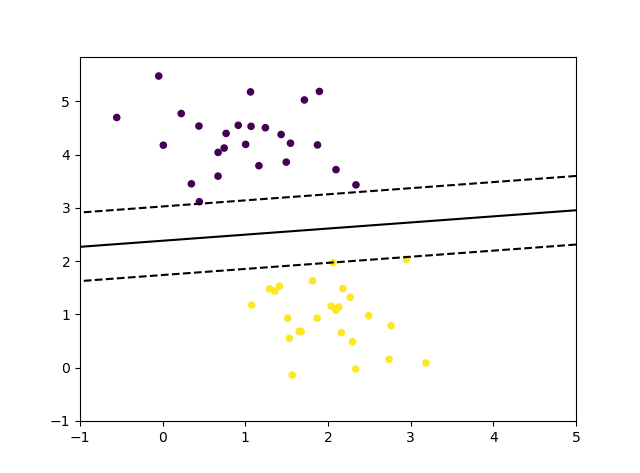随机梯度下降法进行多分类
SGDClassifier 通过利用 “one versus all” （OVA）方法来组合多个二分类器，从而实现多分类。对于每一个 K 类, 可以训练一个二分类器来区分自身和其他 K-1 个类。
在 multi-class classification （多类分类）的情况下， coef_ 是 shape=[n_classes, n_features] 的一个二维数组， intercept_ 是 shape=[n_classes] 的一个一维数组。 coef_ 的第 i 行保存了第 i 类的 OVA 分类器的权重向量；类以升序索引 （参照属性 classes_ ）。 注意，原则上，由于它们允许创建一个概率模型，所以 loss=“log” 和 loss=“modified_huber” 更适合于 one-vs-all 分类。
SGDClassifier 通过拟合参数 class_weight 和 sample_weight 来支持 weighted classes （加权类）和 weighted instances（加权实例）。
# ==============随机梯度下降法进行多分类=============
from sklearn.linear_model import SGDClassifier
from sklearn.metrics import accuracy_score
from sklearn import datasets
X,y=iris.data,iris.target
clf = SGDClassifier(alpha=0.001, max_iter=100).fit(X, y)
y_pred = clf.predict(X)
print('三分类花卉数据准确率：',accuracy_score(y,y_pred))
print('包含的二分类器索引：',clf.classes_)    # one versus all 方法来组合多个二分类器
print('回归系数：',clf.coef_)  # 每一个二分类器的回归系数
print('偏差：',clf.intercept_ ) # 每一个二分类器的偏差

随机梯度下降法回归
SGDRegressor 类实现了一个简单的随机梯度下降学习例程，它支持用不同的损失函数和惩罚来拟合线性回归模型。 SGDRegressor 非常适用于有大量训练样本（>10.000)的回归问题，对于其他问题，我们推荐使用 Ridge ，Lasso ，或 ElasticNet 。
具体的损失函数可以通过 loss 参数设置。 SGDRegressor 支持以下的损失函数:
loss=“squared_loss”: Ordinary least squares（普通最小二乘法）,
loss=“huber”: Huber loss for robust regression（Huber回归）,
loss=“epsilon_insensitive”: linear Support Vector Regression（线性支持向量回归）.
Huber 和 epsilon-insensitive 损失函数可用于 robust regression（鲁棒回归）。不敏感区域的宽度必须通过参数 epsilon 来设定。这个参数取决于目标变量的规模。
SGDRegressor 支持 ASGD（平均随机梯度下降） 作为 SGDClassifier。 均值化可以通过设置 average=True 来启用。
对于利用了 squared loss（平方损失）和 l2 penalty（l2惩罚）的回归，在 Ridge 中提供了另一个采取 averaging strategy（平均策略）的 SGD 变体，其使用了随机平均梯度 (SAG) 算法。
# #===============随机梯度下降法回归===============
from sklearn import linear_model
clf = linear_model.SGDRegressor(loss='squared_loss',penalty='l2',alpha=0.01,max_iter=1000)
clf.fit(X, y)
print('得分：',clf.score(X,y))
print('回归系数：',clf.coef_)
print('偏差：',clf.intercept_ )



展开全文python 机器学习 sklearn
• SGD：基于LoR(逻辑回归)、SGD梯度下降算法对乳腺癌肿瘤(10+1)进行二分类预测(良/恶性) 目录 输出结果 设计思路 核心代码 输出结果 breast-cancer size (683, 11) 训练集情况 2 344 4...
ML之LoR&SGD：基于LoR(逻辑回归)、SGD梯度下降算法对乳腺癌肿瘤(10+1)进行二分类预测(良/恶性)

目录

输出结果

设计思路

核心代码

输出结果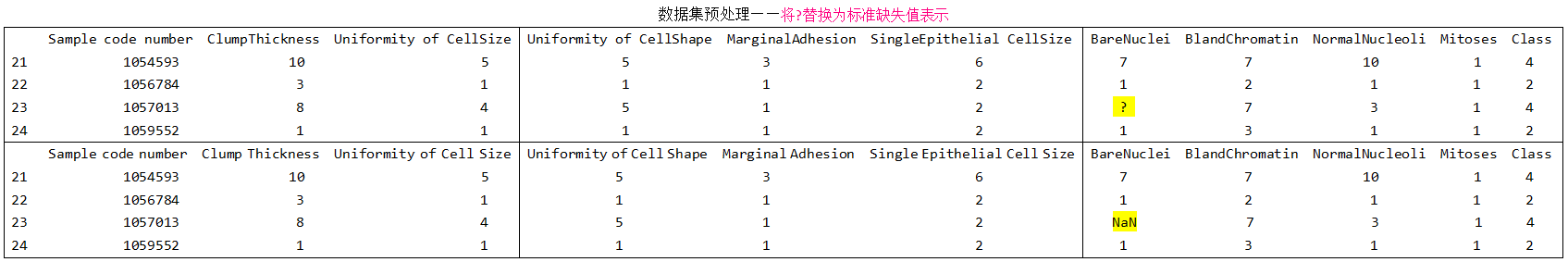breast-cancer size (683, 11)

训练集情况
2    344
4    168
Name: Class, dtype: int64

测试集情况
2    100
4     71
Name: Class, dtype: int64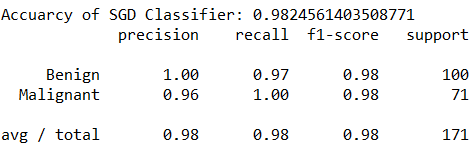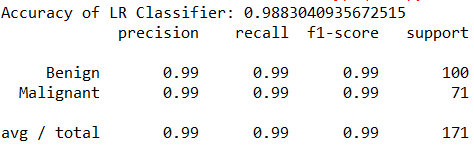设计思路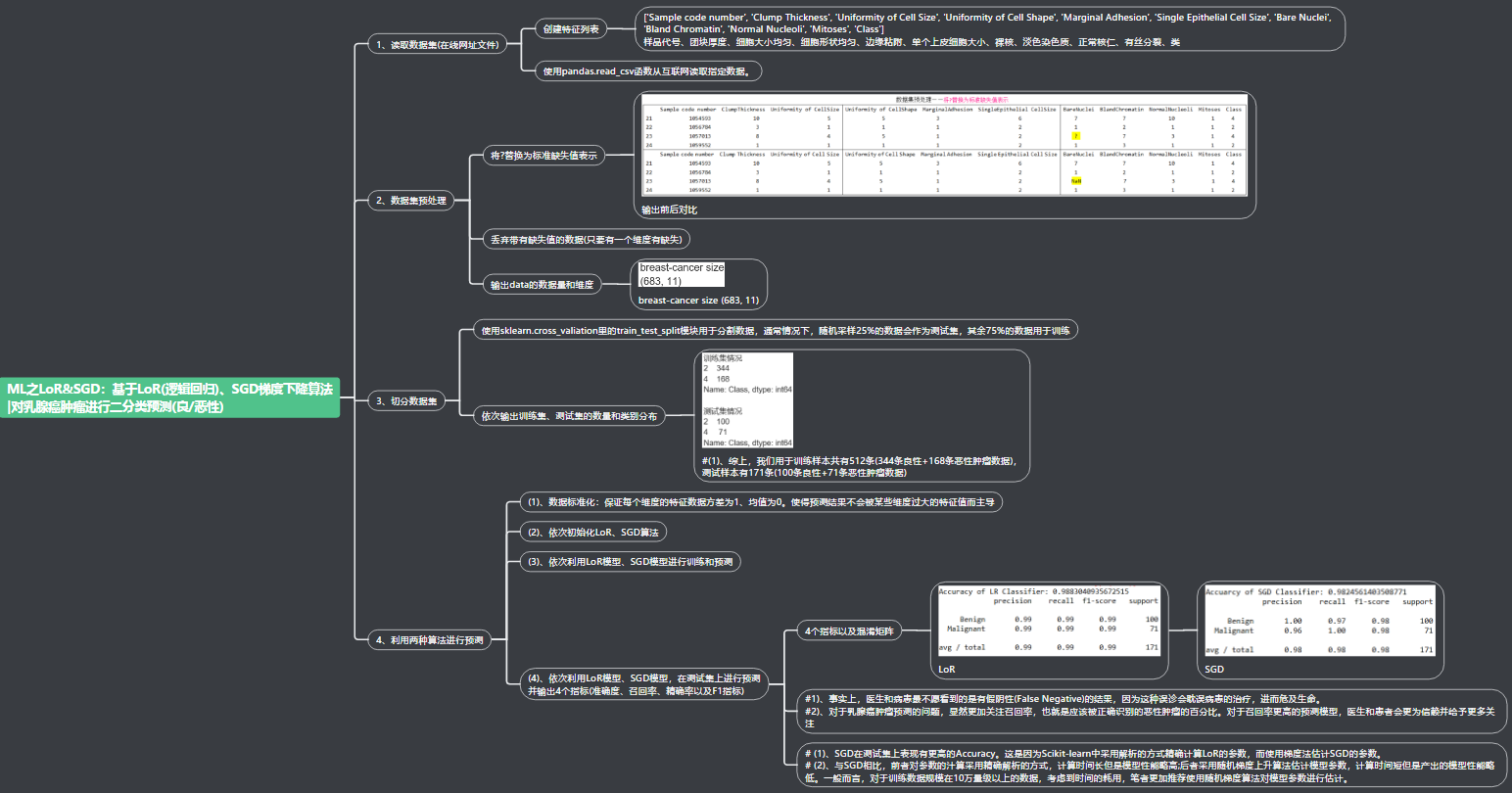核心代码

from sklearn.cross_validation import train_test_split
X_train, X_test, y_train, y_test = train_test_split(data[column_names[1:10]], data[column_names], test_size=0.25, random_state=33)

ss = StandardScaler()
X_train = ss.fit_transform(X_train)
X_test = ss.transform(X_test)

lr = LogisticRegression()
sgdc = SGDClassifier()

lr.fit(X_train, y_train)
lr_y_predict = lr.predict(X_test)

sgdc.fit(X_train, y_train)
sgdc_y_predict = sgdc.predict(X_test)

lr.score(X_test, y_test))
sgdc.score(X_test, y_test))



展开全文• 详细来讲讲梯度下降算法的原理，感受数学和程序的魅力吧！！
博文目录1. 概述2. 梯度下降算法2.1 场景假设2.2 梯度下降2.2.1 微分2.2.2 梯度2.3 数学解释2.3.1 α2.3.2 梯度要乘以一个负号3. 实例3.1 单变量函数的梯度下降3.2 多变量函数的梯度下降4. 代码实现4. 1 场景分析4. 2 代码5. 小结
1. 概述
本文将从一个下山的场景开始，先提出梯度下降算法的基本思想，进而从数学上解释梯度下降算法的原理，解释为什么要用梯度，最后实现一个简单的梯度下降算法的实例！
2. 梯度下降算法
2.1 场景假设
梯度下降法的基本思想可以类比为一个下山的过程。
假设这样一个场景：一个人被困在山上，需要从山上下来(找到山的最低点，也就是山谷)。但此时山上的浓雾很大，导致可视度很低；因此，下山的路径就无法确定，必须利用自己周围的信息一步一步地找到下山的路。这个时候，便可利用梯度下降算法来帮助自己下山。怎么做呢，首先以他当前的所处的位置为基准，寻找这个位置最陡峭的地方，然后朝着下降方向走一步，然后又继续以当前位置为基准，再找最陡峭的地方，再走直到最后到达最低处；同理上山也是如此，只是这时候就变成梯度上升算法了2.2 梯度下降
梯度下降的基本过程就和下山的场景很类似。
首先，我们有一个可微分的函数。这个函数就代表着一座山。我们的目标就是找到这个函数的最小值，也就是山底。根据之前的场景假设，最快的下山的方式就是找到当前位置最陡峭的方向，然后沿着此方向向下走，对应到函数中，就是找到给定点的梯度 ，然后朝着梯度相反的方向，就能让函数值下降的最快！因为梯度的方向就是函数之变化最快的方向(在后面会详细解释)
所以，我们重复利用这个方法，反复求取梯度，最后就能到达局部的最小值，这就类似于我们下山的过程。而求取梯度就确定了最陡峭的方向，也就是场景中测量方向的手段。那么为什么梯度的方向就是最陡峭的方向呢？接下来，我们从微分开始讲起：
2.2.1 微分
看待微分的意义，可以有不同的角度，最常用的两种是：

函数图像中，某点的切线的斜率
函数的变化率
几个微分的例子：

1.单变量的微分，函数只有一个变量时
$\frac{d(x^2)}{dx}=2x$
$\frac{d(-2y^5)}{dy}=-10y^4$
$\frac{d(5-\theta )^2}{d\theta}=-2(5-\theta)$
2.多变量的微分，当函数有多个变量的时候，即分别对每个变量进行求微分
$\frac{\partial}{\partial x}(x^2y^2) = 2xy^2$
$\frac{\partial}{\partial y}(-2y^5+z^2) = -10y^4$
$\frac{\partial}{\partial \theta_{2}}(5\theta_{1} + 2\theta_{2} - 12\theta_{3}) = 2$
$\frac{\partial}{\partial \theta_{2}}(0.55 - (5\theta_{1} + 2\theta_{2} - 12\theta_{3})) = -2$
2.2.2 梯度
梯度实际上就是多变量微分的一般化。
下面这个例子：
$J(\Theta ) = 0.55 - (5\theta_{1} + 2\theta_{2} - 12\theta_{3})$
$\triangledown J(\Theta ) = \left < \frac{\partial J}{\partial \theta_{1}}, \frac{\partial J}{\partial \theta_{2}},\frac{\partial J}{\partial \theta_{3}} \right > =(-5,-2,12)$
我们可以看到，梯度就是分别对每个变量进行微分，然后用逗号分割开，梯度是用<>包括起来，说明梯度其实一个向量。
梯度是微积分中一个很重要的概念，之前提到过梯度的意义

在单变量的函数中，梯度其实就是函数的微分，代表着函数在某个给定点的切线的斜率
在多变量函数中，梯度是一个向量，向量有方向，梯度的方向就指出了函数在给定点的上升最快的方向

这也就说明了为什么我们需要千方百计的求取梯度！我们需要到达山底，就需要在每一步观测到此时最陡峭的地方，梯度就恰巧告诉了我们这个方向。梯度的方向是函数在给定点上升最快的方向，那么梯度的反方向就是函数在给定点下降最快的方向，这正是我们所需要的。所以我们只要沿着梯度的方向一直走，就能走到局部的最低点！
2.3 数学解释
首先给出数学公式：
${\color{Red} \Theta^1} = {\color{Blue} \Theta^0} + {\color{Green} \alpha} {\color{Purple} \triangledown J(\Theta)}\rightarrow evaluated at \Theta^0$
此公式的意义是：J是关于Θ的一个函数，我们当前所处的位置为Θ0点，要从这个点走到J的最小值点，也就是山底。首先我们先确定前进的方向，也就是梯度的反向，然后走一段距离的步长，也就是α，走完这个段步长，就到达了Θ1这个点！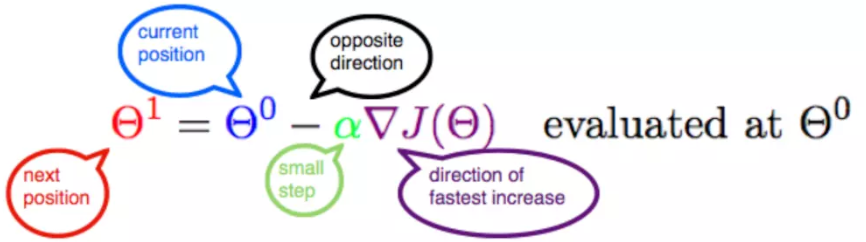2.3.1 α
α在梯度下降算法中被称作为学习率或者步长，意味着我们可以通过α来控制每一步走的距离，以保证不要步子跨的太大扯着蛋，哈哈，其实就是不要走太快，错过了最低点。同时也要保证不要走的太慢，导致太阳下山了，还没有走到山下。所以α的选择在梯度下降法中往往是很重要的！α不能太大也不能太小，太小的话，可能导致迟迟走不到最低点，太大的话，会导致错过最低点！
2.3.2 梯度要乘以一个负号
梯度前加一个负号，就意味着朝着梯度相反的方向前进！我们在前文提到，梯度的方向实际就是函数在此点上升最快的方向！而我们需要朝着下降最快的方向走，自然就是负的梯度的方向，所以此处需要加上负号；那么如果时上坡，也就是梯度上升算法，当然就不需要添加负号了。
3. 实例
我们已经基本了解了梯度下降算法的计算过程，那么我们就来看几个梯度下降算法的小实例，首先从单变量的函数开始，然后介绍多变量的函数。
3.1 单变量函数的梯度下降
我们假设有一个单变量的函数
$J(\theta) = \theta^2$
函数的微分，直接求导就可以得到
$J'(\theta) = 2\theta$
初始化，也就是起点，起点可以随意的设置，这里设置为1
$\theta^0 = 1$
学习率也可以随意的设置，这里设置为0.4
$\alpha = 0.4$
根据梯度下降的计算公式
${\color{Red} \Theta^1} = {\color{Blue} \Theta^0} + {\color{Green} \alpha} {\color{Purple} \triangledown J(\Theta)}\rightarrow evaluated at \Theta^0$
我们开始进行梯度下降的迭代计算过程：
$\theta^0 = 1$
$\theta^1 = \theta^0 - \alpha*J'(\theta^0)=1 - 0.4*2 = 0.2$
$\theta^2 = \theta^1 - \alpha*J'(\theta^1)= 0.2 - 0.4*0.4=0.04$
$\theta^3 = 0.008$
$\theta^4 = 0.0016$
如图，经过四次的运算，也就是走了四步，基本就抵达了函数的最低点，也就是山底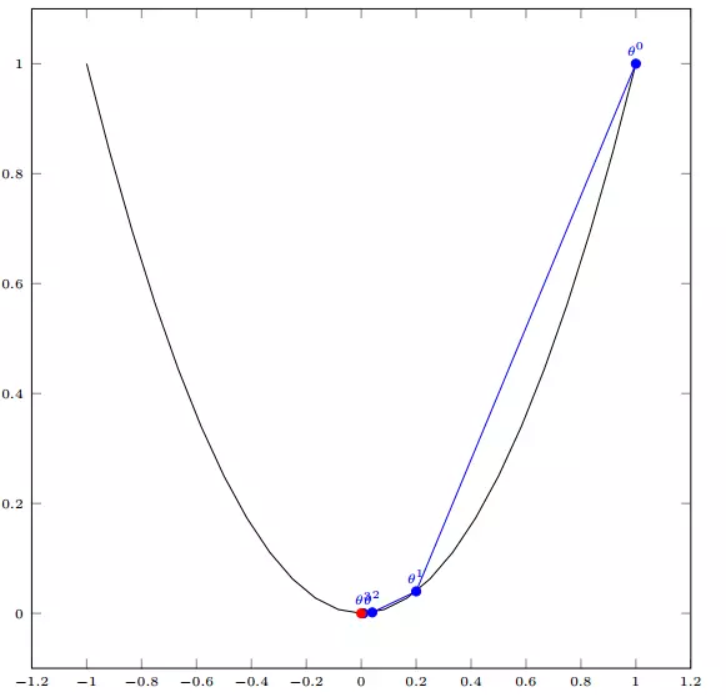3.2 多变量函数的梯度下降
我们假设有一个目标函数
$J(\Theta) = \theta_{1}^2 + \theta_{2}^2$
现在要通过梯度下降法计算这个函数的最小值。我们通过观察就能发现最小值其实就是 (0，0)点。但是接下来，我们会从梯度下降算法开始一步步计算到这个最小值！
我们假设初始的起点为：
$\Theta^0 = (1, 3)$
初始的学习率为：
$\alpha = 0.1$
函数的梯度为：
$\triangledown J(\Theta ) = \left < 2\theta_{1},2\theta_{2} \right >$
进行多次迭代：
$\Theta^0 = (1, 3)$
$\Theta^1 = \Theta^0 - \alpha\triangledown J(\Theta ) = (1,3) - 0.1*(2, 6)=(0.8, 2.4)$
$\Theta^2 = (0.8, 2.4) - 0.1*(1.6, 4.8)=(0.64, 1.92)$
$\Theta^3 =(0.5124, 1.536)$
$\Theta^4 =(0.4096, 1.228800000000001)$
$\vdots$
$\Theta^{10} =(0.1073741824000003, 0.32212254720000005)$
$\vdots$
$\Theta^{50} =(1.141798154164342e^{-05}, 3.42539442494306e^{-05})$
$\vdots$
$\Theta^{100} =(1.6296287810675902e^{-10}, 4.8888886343202771e^{-10})$
我们发现，已经基本靠近函数的最小值点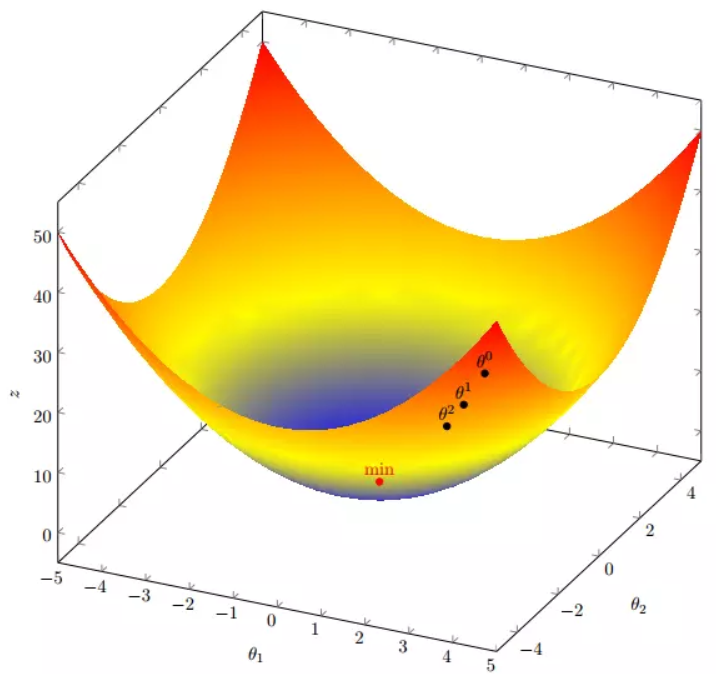4. 代码实现
4. 1 场景分析
下面我们将用python实现一个简单的梯度下降算法。场景是一个简单的线性回归的例子：假设现在我们有一系列的点，如下图所示：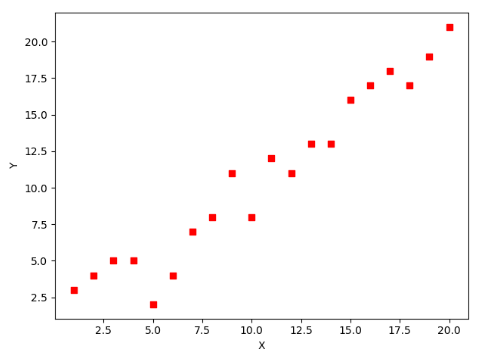我们将用梯度下降法来拟合出这条直线！
首先，我们需要定义一个代价函数，在此我们选用均方误差代价函数（也称平方误差代价函数）
$J(\Theta) = \frac{1}{2m}\sum_{i=1}^{m}(h_{\theta}(x^{(i)})-y^{(i)})^2$
此公式中

m是数据集中数据点的个数，也就是样本数
½是一个常量，这样是为了在求梯度的时候，二次方乘下来的2就和这里的½抵消了，自然就没有多余的常数系数，方便后续的计算，同时对结果不会有影响
y 是数据集中每个点的真实y坐标的值，也就是类标签
h 是我们的预测函数（假设函数），根据每一个输入x，根据Θ 计算得到预测的y值，即

$h_{\Theta}(x^{(i)}) = \Theta_{0} + \Theta_{1}x_{1}^{(i)}$
我们可以根据代价函数看到，代价函数中的变量有两个，所以是一个多变量的梯度下降问题，求解出代价函数的梯度，也就是分别对两个变量进行微分
$\triangledown J(\Theta ) = \left < \frac{\delta J}{\delta \Theta_{0}}, \frac{\delta J}{\delta \Theta_{1}} \right >$
$\frac{\delta J}{\delta \Theta_{0}} = \frac{1}{m}\sum_{i=1}^{m}(h_{\Theta}(x^{(i)})-y^{(i)})$
$\frac{\delta J}{\delta \Theta_{1}} = \frac{1}{m}\sum_{i=1}^{m}(h_{\Theta}(x^{(i)})-y^{(i)})x_{1}^{(i)}$
明确了代价函数和梯度，以及预测的函数形式。我们就可以开始编写代码了。但在这之前，需要说明一点，就是为了方便代码的编写，我们会将所有的公式都转换为矩阵的形式，python中计算矩阵是非常方便的，同时代码也会变得非常的简洁。
为了转换为矩阵的计算，我们观察到预测函数的形式
$h_{\Theta}(x^{(i)}) = \Theta_{0} + \Theta_{1}x^{(i)}$
我们有两个变量，为了对这个公式进行矩阵化，我们可以给每一个点x增加一维，这一维的值固定为1，这一维将会乘到Θ0上。这样就方便我们统一矩阵化的计算
$(x_{1}^{(i)},y^{(i)})\rightarrow (x_{0}^{(i)},x_{1}^{(i)},y^{(i)}) with x_{0}^{(i)} = 1 \forall _{i}$
然后我们将代价函数和梯度转化为矩阵向量相乘的形式
$J(\Theta) = \frac{1}{2m}(X\Theta - \vec{y})^{T}(X\Theta - \vec{y})$
$\triangledown J(\Theta) = \frac{1}{m}X^{T}(X\Theta - \vec{y}))$
4. 2 代码
首先，我们需要定义数据集和学习率
#!/usr/bin/env python3
# -*- coding: utf-8 -*-
# @Time    : 2019/1/21 21:06
# @Author  : Arrow and Bullet
# @Software: PyCharm
# @Blog    ：https://blog.csdn.net/qq_41800366

from numpy import *

# 数据集大小 即20个数据点
m = 20
# x的坐标以及对应的矩阵
X0 = ones((m, 1))  # 生成一个m行1列的向量，也就是x0，全是1
X1 = arange(1, m+1).reshape(m, 1)  # 生成一个m行1列的向量，也就是x1，从1到m
X = hstack((X0, X1))  # 按照列堆叠形成数组，其实就是样本数据
# 对应的y坐标
y = np.array([
3, 4, 5, 5, 2, 4, 7, 8, 11, 8, 12,
11, 13, 13, 16, 17, 18, 17, 19, 21
]).reshape(m, 1)
# 学习率
alpha = 0.01

接下来我们以矩阵向量的形式定义代价函数和代价函数的梯度
# 定义代价函数
def cost_function(theta, X, Y):
diff = dot(X, theta) - Y  # dot() 数组需要像矩阵那样相乘，就需要用到dot()
return (1/(2*m)) * dot(diff.transpose(), diff)

# 定义代价函数对应的梯度函数
diff = dot(X, theta) - Y
return (1/m) * dot(X.transpose(), diff)

最后就是算法的核心部分，梯度下降迭代计算
# 梯度下降迭代
theta = array([1, 1]).reshape(2, 1)
theta = theta - alpha * gradient
return theta

print('optimal:', optimal)
print('cost function:', cost_function(optimal, X, Y))

当梯度小于1e-5时，说明已经进入了比较平滑的状态，类似于山谷的状态，这时候再继续迭代效果也不大了，所以这个时候可以退出循环！
运行代码，计算得到的结果如下：
print('optimal:', optimal)  # 结果 [[0.51583286][0.96992163]]
print('cost function:', cost_function(optimal, X, Y))  # 1.014962406233101

通过matplotlib画出图像，
# 根据数据画出对应的图像
def plot(X, Y, theta):
import matplotlib.pyplot as plt
ax = plt.subplot(111)  # 这是我改的
ax.scatter(X, Y, s=30, c="red", marker="s")
plt.xlabel("X")
plt.ylabel("Y")
x = arange(0, 21, 0.2)  # x的范围
y = theta + theta*x
ax.plot(x, y)
plt.show()

plot(X1, Y, optimal)

所拟合出的直线如下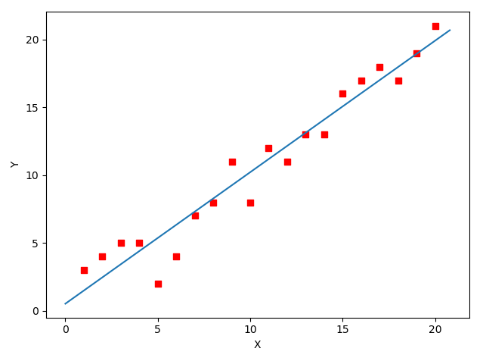全部代码如下，大家有兴趣的可以复制下来跑一下看一下结果：
#!/usr/bin/env python3
# -*- coding: utf-8 -*-
# @Time    : 2019/1/21 21:06
# @Author  : Arrow and Bullet
# @Software: PyCharm
# @Blog    ：https://blog.csdn.net/qq_41800366

from numpy import *

# 数据集大小 即20个数据点
m = 20
# x的坐标以及对应的矩阵
X0 = ones((m, 1))  # 生成一个m行1列的向量，也就是x0，全是1
X1 = arange(1, m+1).reshape(m, 1)  # 生成一个m行1列的向量，也就是x1，从1到m
X = hstack((X0, X1))  # 按照列堆叠形成数组，其实就是样本数据
# 对应的y坐标
Y = array([
3, 4, 5, 5, 2, 4, 7, 8, 11, 8, 12,
11, 13, 13, 16, 17, 18, 17, 19, 21
]).reshape(m, 1)
# 学习率
alpha = 0.01

# 定义代价函数
def cost_function(theta, X, Y):
diff = dot(X, theta) - Y  # dot() 数组需要像矩阵那样相乘，就需要用到dot()
return (1/(2*m)) * dot(diff.transpose(), diff)

# 定义代价函数对应的梯度函数
diff = dot(X, theta) - Y
return (1/m) * dot(X.transpose(), diff)

# 梯度下降迭代
theta = array([1, 1]).reshape(2, 1)
theta = theta - alpha * gradient
return theta

print('optimal:', optimal)
print('cost function:', cost_function(optimal, X, Y))

# 根据数据画出对应的图像
def plot(X, Y, theta):
import matplotlib.pyplot as plt
ax = plt.subplot(111)  # 这是我改的
ax.scatter(X, Y, s=30, c="red", marker="s")
plt.xlabel("X")
plt.ylabel("Y")
x = arange(0, 21, 0.2)  # x的范围
y = theta + theta*x
ax.plot(x, y)
plt.show()

plot(X1, Y, optimal)

5. 小结
至此，就基本介绍完了梯度下降法的基本思想和算法流程，并且用python实现了一个简单的梯度下降算法拟合直线的案例！
最后，我们回到文章开头所提出的场景假设:
这个下山的人实际上就代表了反向传播算法，下山的路径其实就代表着算法中一直在寻找的参数Θ，山上当前点的最陡峭的方向实际上就是代价函数在这一点的梯度方向，场景中观测最陡峭方向所用的工具就是微分 。在下一次观测之前的时间就是有我们算法中的学习率α所定义的。
可以看到场景假设和梯度下降算法很好的完成了对应！
本文部分内容来自一位前辈，非常感谢分享！谢谢！


展开全文• 引言: 在深度学习的任务目标中，通常我们希望我们的学习结果能够在损失函数上得到一个较好的...首先我们知道梯度方向是函数增长最快的方向，梯度的反方向是函数减少最快的方向，而梯度下降法就是往梯度反方向前进...

引言:
在深度学习的任务目标中，通常我们希望我们的学习结果能够在损失函数上得到一个较好的结果，即朝着损失函数最小的方向前进。接下来我会用比较通俗易懂的语言来介绍GD、SGD

1. 数学理解
首先我们知道梯度方向是函数增长最快的方向，梯度的反方向是函数减少最快的方向，而梯度下降法就是往梯度反方向前进"一小步"来达到函数减少的效果。对于二维空间，其下降的方式大致为下图（这里我默认大家都理解等高线）：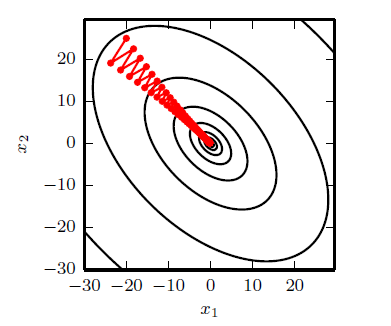接下来，我们来理解为什么是"一小步"，而不是"一大步"?  首先，我们知道，我们一般执行梯度下降是采用下面这个形式：
$x=x-\eta\nabla g$  其中$\eta$就是我们的学习率，$g$是梯度。
我们可以从一元函数的$taylor$公式展开的角度来理解这个问题： $f(x+\eta)\approx f(x)+f' (x)\eta+O(\eta^2)$  其中$f'(x)=\nabla g$。首先，可以从这个近似中（因为我们就只展开了两项，所以$\eta$不能太大）得知$\eta$足够小时才能近似成立，这也就回答了为什么我们要走"一小步"，此外，如果我们把$\eta$换成$-f'(x)\eta$（此时$(x-\eta f'(x)$就是我们常说的往负梯度方向进行更新一小步）然后有
$f(x-\eta f'(x))\approx f(x)\underbrace{-[f'(x)]^2\eta+O(\eta^2)}_{<=0}$  从这里我们可以发现，如果我们往负梯度方向前进一小步，其函数值就会变小，因此可以获得两个我们合乎逻辑的信息：
1. 学习率$\eta$我们一般设为$>=0$的原因  2. 梯度下降法确实能让我们目标函数减小

2. 图画理解从图中我们可以看出，如果学习率较大，很有可能会陷入一个"局部最小值"而无法跳出从图中我们可以看出，如果学习率较小，就需要很多迭代轮数

随机梯度下降法（SGD）的思想就是按照数据生成分布抽取$m$个样本，通过计算他们梯度的平均值来更新梯度（梯度下降法采用的是全部样本的梯度平均值来更新梯度）。
NOTE: 一般来说我们在实现SGD一般采用的都是以上方法，即通过每次取一个batch_size大小的样本来更新梯度而不是每次仅取1个样本来更新。
1. SGD与GD的比较：
在深度学习中，目标函数通常是训练数据集中各个样本的损失函数平均，即$f(x)= \frac{1}{n}\sum_{i=1}^{n}f_i(x)$其中,$f_i(x)$是第i个样本所对应的损失函数，$f(x)$是目标损失函数。
通常，对于梯度下降法而言，其梯度的计算公式为$\nabla f(x)=\frac{1}{n}\sum_{i=1}^{n}\nabla f_i(x)$其计算复杂度为$O(n)$而随机梯度下降法其梯度的计算公式为$\nabla f(x)=\frac{1}{m}\sum_{i=1}^{m}\nabla f_i(x)$其计算复杂度为$O(1)$，因为$m$不会随着$n$的增大而明显改变，这就可以大大减少我们的计算复杂度。
2. batch_size的选择：
一般来说，batch_size也就是$m$一般选择为2的整数次幂。

学习率总结
对于学习率，一般来说我们有如下共识：
1. 较小的学习率意味着收敛速度慢，需要很多的迭代步数；
2.较大的学习率不仅会让前面的$taylor$公式近似不成立，此外也可能因为太大而导致陷入"比较差"的局部最小值

 Ian Goodfellow,Yoshua Bengio,Aaron courville.深度学习[M].人民邮电出版社.
 动手学习深度学习. 李沐

如果觉得我有地方讲的不好的或者有错误的欢迎给我留言，谢谢大家阅读（点个赞我可是会很开心的哦）~


展开全文• 文章目录线性回归学习目标2.5 梯度下降法介绍1 全梯度下降算法（FG）2 随机梯度下降算法（SG）3 小批量梯度下降算法（mini-bantch）4 随机平均梯度下降算法（SAG）5 算法比较6 梯度下降优化算法(拓展) 学习目标 ...python 神经网络 机器学习 人工智能
• bach gd和 mini-bach gd,sgd比较： 参考： https://my.oschina.net/hosee/blog/510076  https://www.zhihu.com/question/40892922?sort=created  https://www.zhihu.com/question/27012077  http
• 阅读目录批量梯度下降法BGD随机梯度下降法SGD小批量梯度下降法MBGD总结 批量梯度下降法BGD 随机梯度下降法SGD 小批量梯度下降法MBGD 总结 在应用机器学习算法时，我们常采用梯度下降法来对才用的算法进行训练。梯度...
• 1. SGD 梯度下降法 1.1 梯度下降（Gradient Descent） 梯度g指函数的某处的偏导数，指向函数上升方向。因此梯度下降法是指用梯度的负数-g更新参数，从而使下一次计算的结果向函数下降方向逼近，从而得到最小值。其中...
• 无论是机器学习（Machine Learning）...下面我们逐个介绍梯度下降法（GD）、随机梯度下降法SGD）和随机平均梯度下降法（SAGD）。先来看梯度下降法的基本思想。 基本原理 如果抛开具体场景，从数学抽象角度来看...
• 随机梯度下降法SGD） 每看一个数据就计算损失函数，然后计算梯度，更新参数 优点：速度快 缺点：虽然速度快，但收敛性能不太好，可能在最优点附近摆动，无法得出最优点；两次参数的更新也可能相互抵消。 批量（微...
• 中心思想：随机梯度下降法是每次先将大量的训练集中随机打乱顺序，再取其中固定数量的实例组成mini_batch，按照minibatch进行权重和偏差的更新，重复操作直到所有的数据都按minibatch的规格取完了一遍，这时就完成了...
• 　在应用机器学习算法时，我们通常采用梯度下降法来对采用的算法进行训练。其实，常用的梯度下降法还具体包含有三种不同的形式，它们也各自有着不同的优缺点。 　下面我们以线性回归算法来对三种梯度下降法进行比较...
• 梯度下降法（BGD）、随机梯度下降法SGD）、小批量梯度下降法（MBGD）之间的关系及batch size如何选取 我们都知道，神经网络在更新权值的时候需要先求得损失函数，再由损失函数求得各参数的梯度进行更新，这里就...神经网络 深度学习
• k_SGD_RECORD.append(k_SGD) #MBGD k_MBGD = 0.0 k_MBGD_RECORD = [] for step in range(TRAIN_STEP): SUM_MBGD = 0 index_start = np.random.randint(len(X_INPUT) - BATCH_SIZE) for index in...python
• 在学习线性回归的时候很多课程都会讲到用梯度下降法求解参数，对于梯度下降算法怎么求出这个解讲的较少，自己实现一遍算法比较有助于理解算法，也能注意到比较细节的东西。具体的数学推导可以参照这一篇博客...python实现
• 日萌社 人工智能AI：Keras PyTorch MXNet TensorFlow PaddlePaddle 深度学习实战（不定时更新） 3. 常见的优化算法介绍 3.1 梯度下降算法（batch gradient descent BGD） ...3.2 随机梯度下降法 (...人工智能
• 沿着负梯度就可以找到最小值，所谓的梯度下降。两者其本质都是一样的。简单公式推导如下：由此可以得到权值更新为（没有引入学习率）：程序具体实现过程：原始数据为A=（M*N）阶矩阵标签为B=（M*1）初始权重为W=（N*...
• 代码： package mllib import org.apache.log4j.{Level, Logger} import org.apache.spark.{SparkContext, SparkConf} import scala.collection.mutable.... * 随机梯度下降算法 * Created by 汪本成 o
• 随机梯度下降(SGD)与经典的梯度下降法的区别 经典的优化方法，例如梯度下降法，在每次迭代过程中需要使用所有的训练数据，这就给求解大规模数据优化问题带来挑战。 知识点：随机梯度下降法(SGD)、小批量梯度下降法。...机器学习
• 梯度下降算法： 代表第i个向量（或者说是输入数据）的第j个元素一个估计出来的模型的表达式h损失函数mse：只是针对一个样本而言的求梯度： 只是针对一个样本而言的梯度递减：为学习效率,也就是梯度下降移动的步长...
• 随机梯度下降算法SGD 参考：为什么说随机最速下降法 (SGD) 是一个很好的方法？ 假如我们要优化一个函数f(x)f(x)f(x) ，即找到它的最小值，常用的方法叫做 Gradient Descent (GD)，也就是最速下降法。说起来很简单, ...神经网络 人工智能 深度学习
• ## 随机梯度下降SGD算法理解

万次阅读 多人点赞 2019-05-29 10:13:21
• 本文基于吴恩达老师的机器学习课程。看了吴恩达老师的机器学习课程，收获很多，想把课上学做的...上一篇博客机器学习（三）：线性回归：梯度下降算法讲了用最小二乘法求得损失函数，再用梯度下降算法最小化损失函数...机器学习
• 1. 批量梯度下降法BGD  批量梯度下降法（Batch Gradient Descent，简称BGD）是梯度下降法最原始的形式，它的具体思路是在更新每一参数时都使用所有的样本来进行更新，其数学形式如下： 　(1) 对上述的能量...
• SGD系列 1）Batch gradient descent(批量梯度下降)   在整个数据集上 ...  随机梯度下降SGD）和批量梯度下降（BGD）的区别。SGD 从数据集中拿出一个样本，并计算相关的误差梯度，而批量梯度下降使用所有......